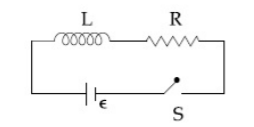####As shown in the figure, a battery of emfis connected to an inductor L and resistance R in series. The switch is closed at t = 0. The total charge that flows from the battery, between t = 0 and t = tc (tc is the time constant of the circuit ) is :  Option: 1Option: 2Option: 3Option: 4As current at any time t is given asSoSo integrating this will give total chargeHence the correct option is (4).